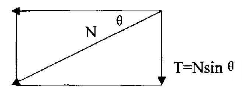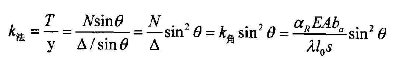# 在GEO5基坑模块中角撑能否考虑？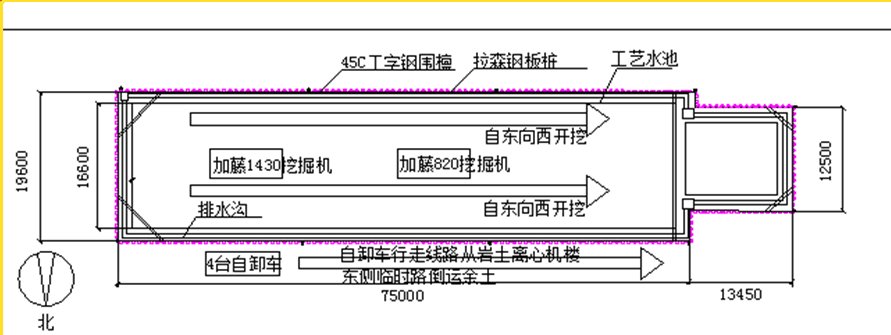• 假设一：角撑只能受轴力作用，即角撑不受弯矩和剪力作用。

• 假设二：角撑不发生旋转，即角撑相对于基坑边的角度不发生变化。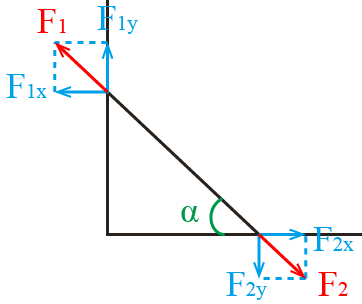F1y和F2x由于平行坑边，对阻止基坑向坑内移动并没有效果，因此，在平面计算中我们得到的支撑反力即为F1x或F2y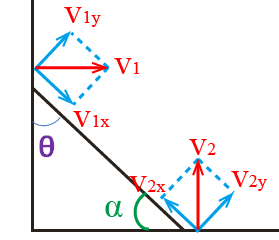F1 =  F1x/cosα = v1x × (EA/L) = v1cosα × (EA/L)

F1x = v1cos2α × (EA/L)

k = (EA/L) × cos2α

• E - 支撑的弹性模量

• A - 支撑的截面面积

• L - 支撑的真实长度

k= （EA/L） X COS2(90°-α） = （EA/L) X sin2θ

• 当v2x = 0 时，λ = 1，即基坑另一侧按固定支座考虑时。

• 当v2x = v1x 时，λ = 0.5，即支撑两对边基坑的土性、深度、宽度、周边荷载等条件相近，且分层对称开挖时。

• 当v2x < v1x 时，0.5 < λ < 1，即计算一侧土压力大于另一侧土压力，或先开挖时。

• 当v2x > v1x 时，0 < λ < 0.5，即计算一侧土压力小于另一侧土压力，或后开挖时。

• 支撑深度：真实深度

• 长度：L/cos2α （如上文所述）

• 支撑水平间距：本例中基坑短边为「宽度/2」，长边为「角撑据基坑阴角距离的两倍」。实际上这里输入的是角撑分担的土压力纵向计算宽度，估算即可。

• 倾角：0°

• 弹性模量：真实弹性模量

• 面积：真实截面面积

• 支撑不动点调整系数：如上文所述

• 支撑松弛系数：如建筑基坑规范所述

• 预应力：真实轴向预应力 × cosα